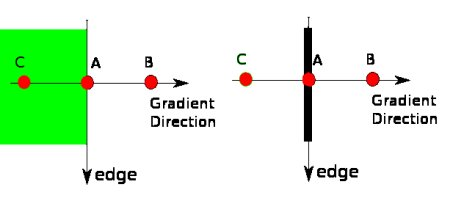# Canny边缘检测

### 理论

Canny Edge Detection是一种流行的边缘检测算法。它由John F. Canny发明

1. 这是一个多阶段算法，我们将经历每个阶段。

2. 降噪

1. 查找图像的强度梯度

$$Edge\_Gradient \; (G) = \sqrt{G_x^2 + G_y^2} \\ Angle \; (\theta) = \tan^{-1} \bigg(\frac{G_y}{G_x}\bigg)$$


1. 非极大值抑制 在获得梯度大小和方向后，将对图像进行全面扫描，以去除可能不构成边缘的所有不需要的像素。为此，在每个像素处，检查像素是否是其在梯度方向上附近的局部最大值。查看下面的图片：点A在边缘（垂直方向）上。渐变方向垂直于边缘。点B和C在梯度方向上。因此，将A点与B点和C点进行检查，看是否形成局部最大值。如果是这样，则考虑将其用于下一阶段，否则将其抑制（置为零）。 简而言之，你得到的结果是带有“细边”的二进制图像。

2. 磁滞阈值

![](http://qiniu.aihubs.net/hysteresis.jpg)


在边缘为长线的假设下，该阶段还消除了小像素噪声。



### OpenCV中的Canny Edge检测

OpenCV将以上所有内容放在单个函数cv.Canny()中。我们将看到如何使用它。第一个参数是我们的输入图像。第二个和第三个参数分别是我们的minValmaxVal。第三个参数是perture_size。它是用于查找图像渐变的Sobel内核的大小。默认情况下为3。最后一个参数是L2gradient，它指定用于查找梯度幅度的方程式。如果为True，则使用上面提到的更精确的公式，否则使用以下函数：$Edge_Gradient \; (G) = |G_x| + |G_y|$。默认情况下，它为False

import numpy as np
import cv2 as cv
from matplotlib import pyplot as plt
edges = cv.Canny(img,100,200)
plt.subplot(121),plt.imshow(img,cmap = 'gray')
plt.title('Original Image'), plt.xticks([]), plt.yticks([])
plt.subplot(122),plt.imshow(edges,cmap = 'gray')
plt.title('Edge Image'), plt.xticks([]), plt.yticks([])
plt.show()


### 附加资源

1. Canny edge detector at Wikipedia：http://en.wikipedia.org/wiki/Canny_edge_detector
2. Canny Edge Detection Tutorial：http://dasl.unlv.edu/daslDrexel/alumni/bGreen/www.pages.drexel.edu/_weg22/can_tut.html by Bill Green, 2002.

### 练习

1. 编写一个小应用程序以找到Canny边缘检测，该检测的阈值可以使用两个跟踪栏进行更改。这样，你可以了解阈值的影响。